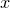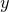# 3.13 Plotting styles

By default, data from files are plotted with points and functions are plotted with lines. However, either kind of data can be plotted in a variety of plot styles. To plot a function with points, for example, the following syntax is used:

```plot sin(x) with points
```

The number of points displayed (i.e. the number of samples of the function) can be set as follows:

```set samples 100
```

Likewise, data files can be plotted with a line connecting the data points:

```plot 'data.dat' with lines
```

A variety of other styles are available. The linespoints plot style combines both the points and lines styles, drawing lines through points. Error bars can also be drawn as follows:

```plot 'data.dat' with yerrorbars
```

In this case, three columns of data need to be specified: the- and-coordinates of each data point, plus the size of the vertical error bar on that data point. By default, the first three columns of the data file are used, but as elsewhere (see Section 3.8), the using modifier can be used:

```plot 'data.dat' using 2:3:7 with yerrorbars
```

Other plot styles supported by Pyxplot are listed in Section 8.2. More details of the errorbars plot style can be found in Section 8.2.2. Bar charts will be discussed in Section 8.2.4.

The modifiers pointtype and linetype, which can be abbreviated to pt and lt respectively, can also be placed after the with modifier. Each should be followed by an integer. The former specifies what shape of points should be used to plot the dataset, and the latter whether a line should be continuous, dotted, dash-dotted, etc. Different integers correspond to different styles, and are listed in Chapter 18.

The default plotting style, used when none is specified to the plot command, can also be changed. For example:

```set style data lines
```

would change the default style used for plotting data from files to lines. Similarly, the set style function command changes the default style used when functions are plotted.